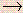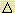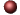Electrochemistry Examples ofMultiple Choice Questions

1.
In an electrolytic cell the electrode at which the electrons enter the solution is called the ______ ; the chemical change that occurs at this electrode is called _______.
(a) anode, oxidation
(b) anode, reduction
(c) cathode, oxidation
(d) cathode, reduction
(e) cannot tell unless we know the species being oxidized and reduced.

2.
Which of the following statements is FALSE?
(a) Oxidation and reduction half-reactions occur at electrodes in electrochemical cells.
(b) All electrochemical reactions involve the transfer of electrons.
(c) Reduction occurs at the cathode.
(d) Oxidation occurs at the anode.
(e) All voltaic (galvanic) cells involve the use of electricity to initiate nonspontaneous chemical reactions.

3.
The half-reaction that occurs at the anode during the electrolysis of molten sodium bromide is:
(a) 2 Br-Br2 + 2 e-
(b) Br2 + 2 e-2 Br-
(c) Na+ + e-Na
(d) NaNa+ + e-
(e) 2 H2O + 2 e-2 OH- + H2

4.
During the electrolysis of aqueous KCl solution using inert electrodes, gaseous hydrogen is evolved at one electrode and gaseous chlorine at the other electrode. The solution around the electrode at which hydrogen gas is evolved becomes basic as the electrolysis proceeds. Which of the following responses describe or are applicable to the cathode and the reaction that occurs at the cathode?
 (1) the positive electrode (2) the negative electrode (3) 2 Cl-Cl2 + 2 e- (4) Cl2 + 2 e-2 Cl- (5) 2 H2OO2 + 4 H+ + 4 e- (6) 2 H2O + 2 e-H2 + 2 OH- (7) electrons flow from the electrode to the external circuit (8) electrons flow into the electrode from the external circuit (9) oxidation (10) reduction

(a) 2, 6, 8, 9
(b) 1, 5, 7, 9
(c) 2, 5, 7, 9
(d) 1, 6, 8, 10
(e) 2, 6, 8, 10

5.
What mass (in grams) of nickel could be electroplated from a solution of nickel(II) chloride by a current of 0.25 amperes flowing for 10 hours?
(a) 12 g
(b) 5.5 g
(c) 0.046 g
(d) 2.7 g
(e) 6.0 g

6.
Molten AlCl3 is electrolyzed for 5.0 hours with a current of 0.40 amperes. Metallic aluminum is produced at one electrode and chlorine gas, Cl2, is produced at the other. How many liters of Cl2 measured at STP are produced when the electrode efficiency is only 65%?
(a) 0.55 L
(b) 0.63 L
(c) 0.84 L
(d) 0.98 L
(e) 1.02 L

7.
How long (in hours) must a current of 5.0 amperes be maintained to electroplate 60 g of calcium from molten CaCl2?
(a) 27 hours
(b) 8.3 hours
(c) 11 hours
(d) 16 hours
(e) 5.9 hours

8.
How long, in hours, would be required for the electroplating of 78 g of platinum from a solution of [PtCl6]2-, using an average current of 10 amperes at an 80% electrode efficiency?
(a) 8.4
(b) 5.4
(c) 16.8
(d) 11.2
(e) 12.4

9.
How many faradays are required to reduce 1.00 g of aluminum(III) to the aluminum metal?
(a) 1.00
(b) 1.50
(c) 3.00
(d) 0.111
(e) 0.250

10.
Which of the following is the strongest oxidizing agent?
(a) Pb2+
(b) I2
(c) Ag+
(d) Pb
(e) Cu2+

11.
As the cell given below operates, the strip of silver gains mass (only silver) and the concentration of silver ions in the solution around the silver strip decreases, while the strip of lead loses mass and the concentration of lead increases in the solution around the lead strip. Which of the following represents the reaction that occurs at the negative electrode in the above cell?
Pb / Pb(NO3)2 (1.0 M) || AgNO3 (1.0 M) / Ag

(a) Pb2+ + 2 e-Pb
(b) PbPb2+ + 2 e-
(c) Ag+ + e-Ag
(d) AgAg+ + e-
(e) none of the above

12.
For a voltaic (or galvanic) cell using Ag,Ag+ (1.0 M) and Zn,Zn2+ (1.0 M) half-cells, which of the following statements is incorrect?
(a) The zinc electrode is the anode.
(b) Electrons will flow through the external circuit from the zinc electrode to the silver electrode.
(c) Reduction occurs at the zinc electrode as the cell operates.
(d) The mass of the zinc electrode will decrease as the cell operates.
(e) The concentration of Ag+ will decrease as the cell operates.

13.
Consider the standard voltaic (or galvanic) cell: Fe,Fe2+ versus Au,Au3+. Which answer identifies the cathode and gives the Eo for the cell?
(a) Fe, -0.44 V
(b) Au, 1.94 V
(c) Fe, 1.06 V
(d) Au, 1.06 V
(e) Fe, 1.94 V

14.
What is the reduction potential for the half-reaction at 25o C:
Al3+ + 3e-Al, if [Al3+] = 0.10 M and Eo = -1.66 V ?
(a) -1.84 V
(b) -1.60 V
(c) -1.68 V
(d) -1.66 V
(e) -1.72 V

15.
What is the value of E for the half-cell:
MnO4- (0.010 M) + 8H+ (0.20 M) + 5e-Mn2+ (0.020 M) + 4H2O ?
(a) 1.50 V
(b) 1.86 V
(c) 1.44 V
(d) 1.58 V
(e) 1.52 V

16.
Calculate the potential (in volts) for the voltaic (or galvanic) cell indicated at 25oC.
Ga / Ga3+ (10-6 M) || Ag+ (10-4 M) / Ag
(a) 1.29 V
(b) 0.97 V
(c) 1.45 V
(d) 1.21 V
(e) 1.37 V

17.
A concentration cell is constructed by placing identical Cu electrodes in two Cu2+ solutions. If the concentrations of the two Cu2+ solutions are 1.0 M and 0.0020 M, calculate the potential of the cell.
(a) 0.020 V
(b) 1.2 V
(c) 0.030 V
(d) 1.0 V
(e) 0.080 V

18.
What isGo per mole of dichromate ions for the reduction of dichromate ions, Cr2O72-, to Cr3+ by bromide ions, Br-, in acidic solution? (Hint: Use the standard cell potential.)
(a) +26.3 kJ
(b) -145 kJ
(c) +145 kJ
(d) -26.3 kJ
(e) -53.6 kJ

19.
Estimate the equilibrium constant for the system indicated at 25oC.
3 Mg2+ + 2Al3Mg + 2Al3+
(a) ~1069
(b) ~1023
(c) ~10-24
(d) ~10-36
(e) ~10-72

20.
In voltaic cells, such as those diagrammed in your text, the salt bridge _______ .
(a) is not necessary in order for the cell to work
(b) acts as a mechanism to allow mechanical mixing of the solutions
(c) allows charge balance to be maintained in the cell
(d) is tightly plugged with firm agar gel through which ions cannot pass
(e) drives free electrons from one half-cell to the other

21.
Which of the following statements is(are) true for all voltaic (or galvanic) cells?
(I) Reduction occurs at the cathode.
(II) The anode gains mass during discharge (note: this means operation of the cell.)
(III) The voltage is less than or equal to zero.

(a) only III
(b) only II
(c) only I
(d) II and III
(e) I, II, and III

22.
In the standard notation for a voltaic cell, the double vertical line "||" represents:
(a) a phase boundary
(b) gas electrode
(c) a wire (metal) connection
(d) a salt bridge
(e) a standard hydrogen electrode

1. (d) 2. (e) 3. (a) 4. (e) 5. (d) 6. (a) 7. (d) 8. (b) 9. (d) 10. (c) 11. (b) 12. (c) 13. (b) 14. (c) 15. (c) 16. (d) 17. (e) 18. (b) 19. (e) 20. (c) 21. (c) 22. (d)Click here to return to the top.Choose your next chapter:
Fundamentals of Chemistry | Chemical Formulas & Composition Stoichiometry | Chemical Equations & Rxn Stoichiometry | Types of Chemical Reactions |
| Atomic Structure | Chemical Periodicity | Chemical Bonding | Molecular Structure/Covalent Bonding Theories| Molecular Orbital Theory |
| Acids/Bases/Salts - Theory & Rxns | Acids/Bases/Salts - Calculations (including balancing redox rxns) | Gases | Solids & Liquids | Solutions |
| Thermodynamics | Kinetics | Equilibrium | Aqueous Equilibrium - Acids/Bases/Salts | Aqueous Equilibrium - Buffers & Titrations |
| Aqueous Equilibrium - Slightly Soluble Salts | Electrochemistry | Metallurgy | Metal Properties & Rxns | Nonmetals & Metalloids |
| Coordination Compounds | Nuclear Chemistry | Organic Chem - Formulas/Names/Properties | Organic Chem - Shapes/Rxns/Biopolymers |To report any corrections, please e-mail Dr. Wendy Keeney-Kennicutt.